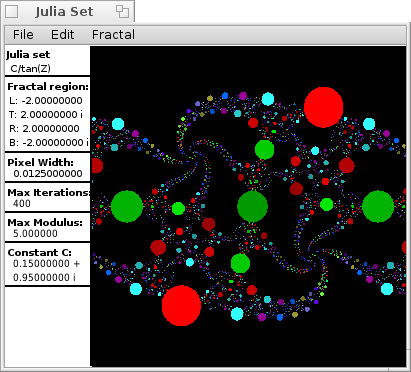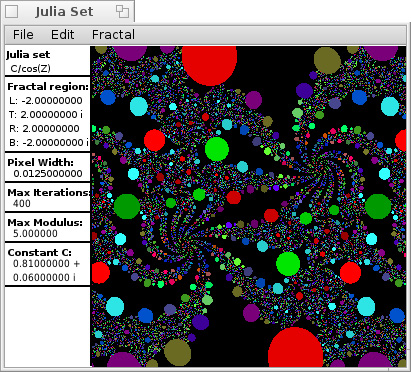Julia Sets Last update=12 Mar, 2022Download JuliaSets, 32-bit executable (updated Mar 12, 2022) Download JuliaSets, 64-bit executable (updated Mar 12, 2022) Some screenshots of various fractals are shown, including some Julia sets and the Mandelbrot set. JuliaSets is software that calculates these. As this is computationally intensive, eight simultaneous threads are used. It is advantageous to have a computer with multiple cores.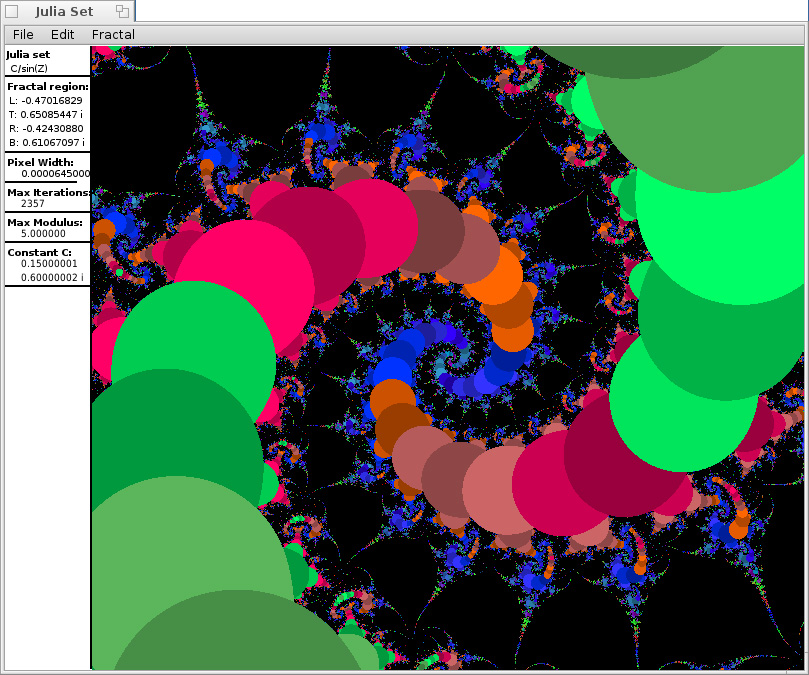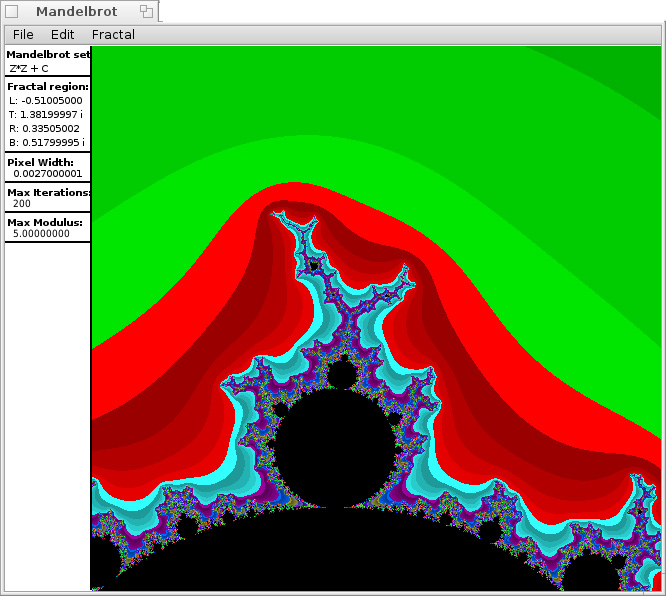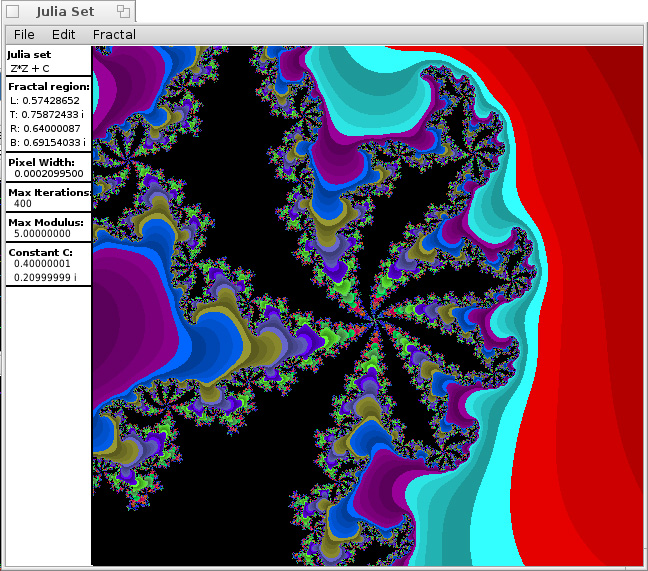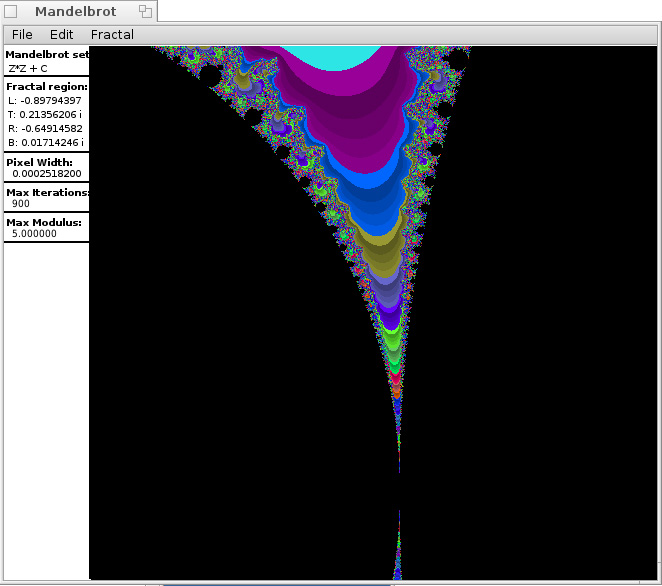These images are constructed as follows. A function f(z) of a complex variable z is chosen, eg. f(z)=z2+c, where c is a constant. A starting value z0 is chosen, and the function is iterated. z1 = f(z0) z2 = f(z1) z3 = f(z2), etc. There are two possible outcomes, either |zn| remains bounded as n → ∞, or else |zn| → ∞ as n → ∞. The value z0 corresponds to a point of the complex plane. If |zn| is unbounded, then z0 belongs to the escape set of the function f(z). But if |zn| is bounded, then z0 belongs to the prisoner set. The Julia set is the boundary of the prisoner set, those points where the prisoner set meets the escape set. This is where the qualitative behaviour of the function changes. In practice it is difficult to determine the Julia set for most functions f(z). A region of the complex plane is chosen, eg., the square region with corners [-2+2i, 2-2i]. A bitmap is constructed for this region, with each pixel in the bitmap corresponding to a point of the complex plane belonging to the region. A maximum modulus M > 0 is chosen, and a maximum number N of iterations is chosen. The function is iterated beginning from each complex z0 which corresponds to a pixel of the bitmap. If after N iterations, the value |zn| < M, it is assumed that the iterations will be bounded. z0 is assumed to be in the prisoner set, and the pixel corresponding to z0 is coloured black. But if |zn| > M after n iterations, where n < N, it is assumed that the iterations will be unbounded, ie., that z0 is in the escape set. The pixel corresponding to z0 is then assigned a colour according to n. For the Mandelbrot set, we always take z0=0, and the constant c is varied within the region of the complex plane. Then c is in the Mandelbrot set if the iterations are bounded. For the Julia sets, c is fixed, and z0 is varied within the region. Braverman and Cook [Notices of the AMS 53, #3, March 2006, "Computing over the reals: foundations for scientific computing"] suggest that the Mandelbrot set could be used as a foundation for a rigorous theory of computing with floating point numbers. They suggest that every program that constructs the Mandelbrot set must have some incorrect pixels. The sofware JuliaSets constructs the Mandelbrot set, as well as Julia sets for various functions f(z). The constant c can be varied, as can M and N, and it can zoom in to see finer and finer detail.